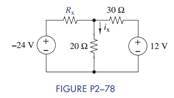Chat Now

# Select a value for R x so that i x = 0 A in Figure P2−78.

### Select a value for R x so that i x = 0 A in Figure P2−78.

Select a value for Rx so that ix = 0 A in Figure P2−78.manish jayant 28-Jul-2020Get solution